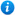# Subtracting Decimals Within 1 - Year 5

Subtracting Decimals Within 1  - Year 5

Year 5 maths programme of study - Number - fractions (including decimals)

• solve problems involving number up to three decimal places

Year 5 maths programme of study - Measurement

• use all four operations to solve problems involving measure using decimal notation

'Subtracting Decimals Within 1  - Year 5' is a KS2 maths teaching resource ideal to use in a lesson covering the year 5 curriculum objective listed above. Content includes:

• Subtracting decimals within 1 using place value charts activities and worksheets x 2
• Subtracting decimals within 1 using a hundred square grid activity and worksheet
• Subtracting decimals within 1 using column subtraction activity and worksheet
• A further problem solving activity and worksheet

'Subtracting Decimals Within 1 - Year 5' is fully editable so teachers are able to adapt the resource to suit the needs of each class they teach.

'Subtracting Decimals Within 1 - Year 5' can be previewed in full by clicking on the PowerPoint images.

Our Price : £2.50 / 3 Credits